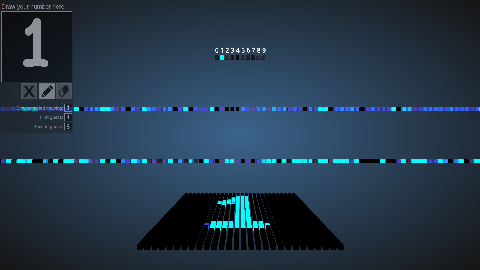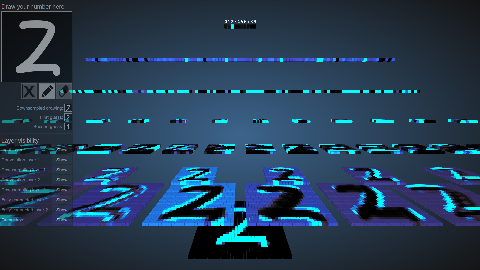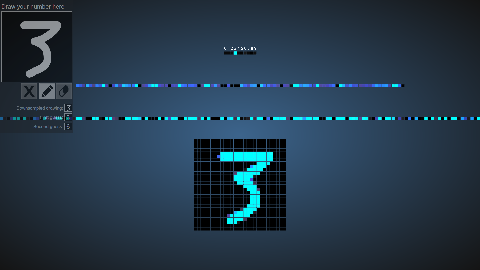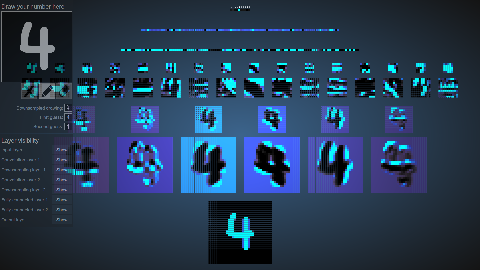# An Interactive Node-Link Visualization of Convolutional Neural Networks

## Abstract

Convolutional neural networks are at the core of state-of-the-art approaches to a variety of computer vision tasks. Visualizations of neural networks typically take the form of static node-link diagrams, which illustrate only the structure of a network, rather than the behavior. Motivated by this observation, this paper presents a new interactive visualization of neural networks trained on handwritten digit recognition, with the intent of showing the actual behavior of the network given user-provided input. The user can interact with the network through a drawing pad, and watch the activation patterns of the network respond in real time.

## Citation

A. W. Harley, "An Interactive Node-Link Visualization of Convolutional Neural Networks," in ISVC, pages 867-877, 2015

Bibtex format:

``` @inproceedings{harley2015isvc,     title = {An Interactive Node-Link Visualization of Convolutional Neural Networks},     author = {Adam W Harley},     booktitle = {ISVC},     pages = {867--877},     year = {2015} } ```

## Demo3D fully-connected network visualization This network has 784 nodes on the bottom layer (corresponding to pixels), 300 nodes in the first hidden layer, 100 nodes in the second hidden layer, and 10 nodes in the output layer (corresponding to the 10 digits).3D convolutional network visualization This network has 1024 nodes on the bottom layer (corresponding to pixels), six 5x5 (stride 1) convolutional filters in the first hidden layer, followed by sixteen 5x5 (stride 1) convolutional filters in the second hidden layer, then three fully-connected layers, with 120 nodes in the first, 100 nodes in the second, and 10 nodes in the third. The convolutional layers are each followed by downsampling layer that does 2x2 max pooling (with stride 2).2D fully-connected network visualization This is the same as the first visualization, but with the nodes flattened on a plane so that they are easier to see all at once.2D convolutional network visualization This is the same as the second visualization, but with the nodes flattened on a plane so that they are easier to see all at once.

## Details

The networks were trained on an augmented version of MNIST, so they excel at categorizing centred upright numbers. The networks were trained in a custom neural network implementation in MATLAB; the math for the visualizations was written in Javascript; the visualization was created in WebGL. The source code for both visualizations is available here.